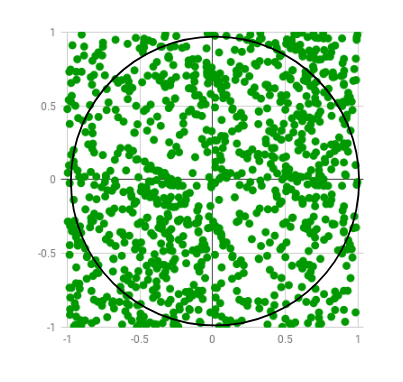# Estimating the value of Pi using Monte Carlo

Monte Carlo estimation
Monte Carlo methods are a broad class of computational algorithms that rely on repeated random sampling to obtain numerical results. One of the basic examples of getting started with the Monte Carlo algorithm is the estimation of Pi.

Estimation of Pi
The idea is to simulate random (x, y) points in a 2-D plane with domain as a square of side 1 unit. Imagine a circle inside the same domain with same diameter and inscribed into the square. We then calculate the ratio of number points that lied inside the circle and total number of generated points. Refer to the image below:Random points are generated only few of which lie outside the imaginary circle

We know that area of the square is 1 unit sq while that of circle is. Now for a very large number of generated points,that is,The beauty of this algorithm is that we don’t need any graphics or simulation to display the generated points. We simply generate random (x, y) pairs and then check if. If yes, we increment the number of points that appears inside the circle. In randomized and simulation algorithms like Monte Carlo, the more the number of iterations, the more accurate the result is. Thus, the title is “Estimating the value of Pi” and not “Calculating the value of Pi”. Below is the algorithm for the method:

The Algorithm
1. Initialize circle_points, square_points and interval to 0.
2. Generate random point x.
3. Generate random point y.
4. Calculate d = x*x + y*y.
5. If d <= 1, increment circle_points.
6. Increment square_points.
7. Increment interval.
8. If increment < NO_OF_ITERATIONS, repeat from 2.
9. Calculate pi = 4*(circle_points/square_points).
10. Terminate.

The code doesn't wait for any input via stdin as the macro INTERVAL could be changed as per the required number of iterations. Number of iterations are the square of INTERVAL. Also, I've paused the screen for first 10 iterations with getch() and outputs are displayed for every iteration with format given below. You can change or delete them as per requirement.

x y circle_points square_points - pi


Examples:


INTERVAL = 5
Output : Final Estimation of Pi = 2.56

INTERVAL = 10
Output : Final Estimation of Pi = 3.24

INTERVAL = 100
Output : Final Estimation of Pi = 3.0916


## C++

 /* C++ program for estimation of Pi using Monte     Carlo Simulation */ #include     // Defines precision for x and y values. More the  // interval, more the number of significant digits  #define INTERVAL 10000  using namespace std;     int main()  {      int interval, i;      double rand_x, rand_y, origin_dist, pi;      int circle_points = 0, square_points = 0;         // Initializing rand()      srand(time(NULL));         // Total Random numbers generated = possible x      // values * possible y values      for (i = 0; i < (INTERVAL * INTERVAL); i++) {             // Randomly generated x and y values          rand_x = double(rand() % (INTERVAL + 1)) / INTERVAL;          rand_y = double(rand() % (INTERVAL + 1)) / INTERVAL;             // Distance between (x, y) from the origin          origin_dist = rand_x * rand_x + rand_y * rand_y;             // Checking if (x, y) lies inside the define          // circle with R=1          if (origin_dist <= 1)              circle_points++;             // Total number of points generated          square_points++;             // estimated pi after this iteration          pi = double(4 * circle_points) / square_points;             // For visual understanding (Optional)          cout << rand_x << " " << rand_y << " " << circle_points               << " " << square_points << " - " << pi << endl << endl;             // Pausing estimation for first 10 values (Optional)          if (i < 20)              getchar();      }         // Final Estimated Value      cout << "\nFinal Estimation of Pi = " << pi;         return 0;  }

## Python

 import random     INTERVAL= 1000    circle_points= 0 square_points= 0    # Total Random numbers generated= possible x  # values* possible y values  for i in range(INTERVAL**2):         # Randomly generated x and y values from a      # uniform distribution      # Rannge of x and y values is -1 to 1      rand_x= random.uniform(-1, 1)      rand_y= random.uniform(-1, 1)         # Distance between (x, y) from the origin      origin_dist= rand_x**2 + rand_y**2        # Checking if (x, y) lies inside the circle      if origin_dist<= 1:          circle_points+= 1        square_points+= 1        # Estimating value of pi,      # pi= 4*(no. of points generated inside the       # circle)/ (no. of points generated inside the square)      pi = 4* circle_points/ square_points     ##    print(rand_x, rand_y, circle_points, square_points, "-", pi)  ##    print("\n")     print("Final Estimation of Pi=", pi)

Output:

Final Estimation of Pi = 3.16116


This article is contributed by Paras Lehana. If you like GeeksforGeeks and would like to contribute, you can also write an article using contribute.geeksforgeeks.org or mail your article to contribute@geeksforgeeks.org. See your article appearing on the GeeksforGeeks main page and help other Geeks.

Attention reader! Don’t stop learning now. Get hold of all the important DSA concepts with the DSA Self Paced Course at a student-friendly price and become industry ready.

My Personal Notes arrow_drop_up

Improved By : MohitBansal3

Article Tags :
Practice Tags :

5

Please write to us at contribute@geeksforgeeks.org to report any issue with the above content.